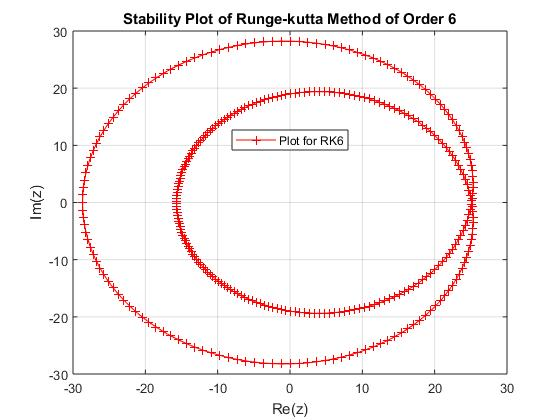# Linear Stability Analysis of Runge-Kutta Methods for Singular Lane-Emden Equations

• M. O. Ogunniran Department of Mathematical Sciences, Osun State University, Osogbo
• O. A. Tayo Department of Mathematical Sciences, Osun State University, Osogbo
• Y. Haruna Department of Mathematics, Saadatu Rimi College of Education Kumbotso, Kano State
• A. F. Adebisi Department of Mathematical Sciences, Osun State University, Osogbo
Keywords: Classical, Stability, Explicit, Lane-Emden equations, Ordinary Differential Equations

### Abstract

Runge-Kutta methods are efficient methods of computations in differential equations, the classical Runge-Kutta method of order 4 happens to be the most popular of these methods, and most times it is attached to the mind when Runge-Kutta methods are mentioned. However, there are numerous forms of them existing in lower and higher orders of the classical method. This work investigates the linear stabilities and abilities of some selected explicit members of these Runge-Kutta methods in integrating the singular Lane-Emden differential equations. The results obtained established the ability of the classical Runge-Kutta method and why is mostly used in computations.

### References

A. Huta, “Contribution `a la formule de sixieme ordre dans la methode de Runge-kutta-Nystr¨om”, Acta Fac Rerum Natu Univ. Comenian Math. 2 (1957) 21.

R. Qu & R. P. Agarwal, “A collocation method for solving a class of singular non-linear two-point boundary value problems”, Journal Computer Application Mathematics 83 (1997) 147.

O. Koch, K. Peter & B. W. Ewa, “The Implicit Euler method for the numerical solution of singular initial value problems”, Applied Numerical Mathematics 34 (2000) 231

A. M. Wazwaz, “A new algorithm for solving differential equation of Lane-Emden type”, Applied Mathematics and Computation 118 (2001) 287.

A. M. Wazwaz, “Adomian decomposition method for a reliable treatment of the Emden-Fowler equation”, Applied Mathematics and Computation 61 (2005) 543.

H. Musa, S. Ibrahim & M. Y. Waziri, “A Simplified Derivation and Analysis of Fourth Order Runge Kutta Method”, International Journal of Computer Applications 9 (2010) 51.

P. Roul, “A new efficient recursive technique for solving singular boundary value problems arising in various physical models”, The European Physical Journal Plus 131 (2016), doi.org/10.1140/epjp/i2016-16105-8.

A. Abbas Al-Shimmary, “Solving Initial Value Problem Using Rungekutta 6th Order Method”, ARPN Journal of Engineering and Applied Sciences 12 (2017) 3953.

P. Roul & K. Thula, “A fourth order B-spline collocation method and its error analysis for Bratu-type and Lane-Emden problems”, International Journal of Computer Mathematics 96 (2017) 85.

H. Madduri, & P. Roul, “A fast-converging iterative scheme for solving a system of Lane–Emden equations arising in catalytic diffusion reactions”, Journal of Mathematical Chemistry 57 (2018) 570.

P. Roul, “Doubly singular boundary value problems with derivative dependent source function: A fast converging iterative approach”, Journal of Math Methods Application Science 42 (2018) 354.

M. O. Ogunniran, Y. Haruna, & R. B. Adeniyi, “Effcient k-derivative Methods for Lane-Emden Equations and Related Stiff Problems”, Nigerian Journal of Mathematics and Applications 28 (2019) 1.

M. O. Ogunniran & R. B. Adeniyi, “New Basic Rational Approximation Method for Solving Singular Initial Value Problems of Ordinary Differential Equations”, ABACUS (Mathematics Science Series) 46 (2019) 119.

M. O. Ogunniran, “A Class of Block Multi-Derivative Numerical Integrator for Singular Advection Equations”, Journal of the Nigerian Society of Physical Sciences 1 (2019) 62.

M. O. Olayiwola, “Solutions of Emden-Fowler Type Equations by Variational Iteration Method”, Cankaya University Journal of Science and Engineering 16 (2019) 1.

M. O. Olayiwola & A. Adegoke, “Homotopy Perturbation with Laplace Transforms Method for Solving Singular Initial Value Problems” Nigerian Journal of Mathematics and Applications 28 (2019) 65.

P. Roul, K. Thula & V. Goura, “An optimal sixth-order quartic B-spline collocation method for solving Bratu-type and Lane-Emden–type problems”, Journal of Computational and Applied Mathematics 359 (2019) DOI: https://doi.org/10.1002/mma.5537

P. Roul, H. Madduri&R. Agarwal, “A fast-converging recursive approach for Lane-Emden type initial value problems arising in astrophysics”, Journal of Math Methods Application Science 42 (2019) 2613.

G. Singh, A. Garg, V. Kanwar & H. Ramos, “An Efficient Optimized Adaptive step-size Hybrid Block Method for Integrating Differential Systems”, Applied Mathematics and Computation, 362 (2019) 124567.

J. D. Lambert, Computational Methods in Ordinary Differential Equations, John Wiley and Sons, New York, 1991.Published
2020-08-01
How to Cite
Ogunniran, M. O., Tayo, O. A., Haruna, Y., & Adebisi, A. F. (2020). Linear Stability Analysis of Runge-Kutta Methods for Singular Lane-Emden Equations. Journal of the Nigerian Society of Physical Sciences, 2(3), 134-140. https://doi.org/10.46481/jnsps.2020.87
Section
Original Research